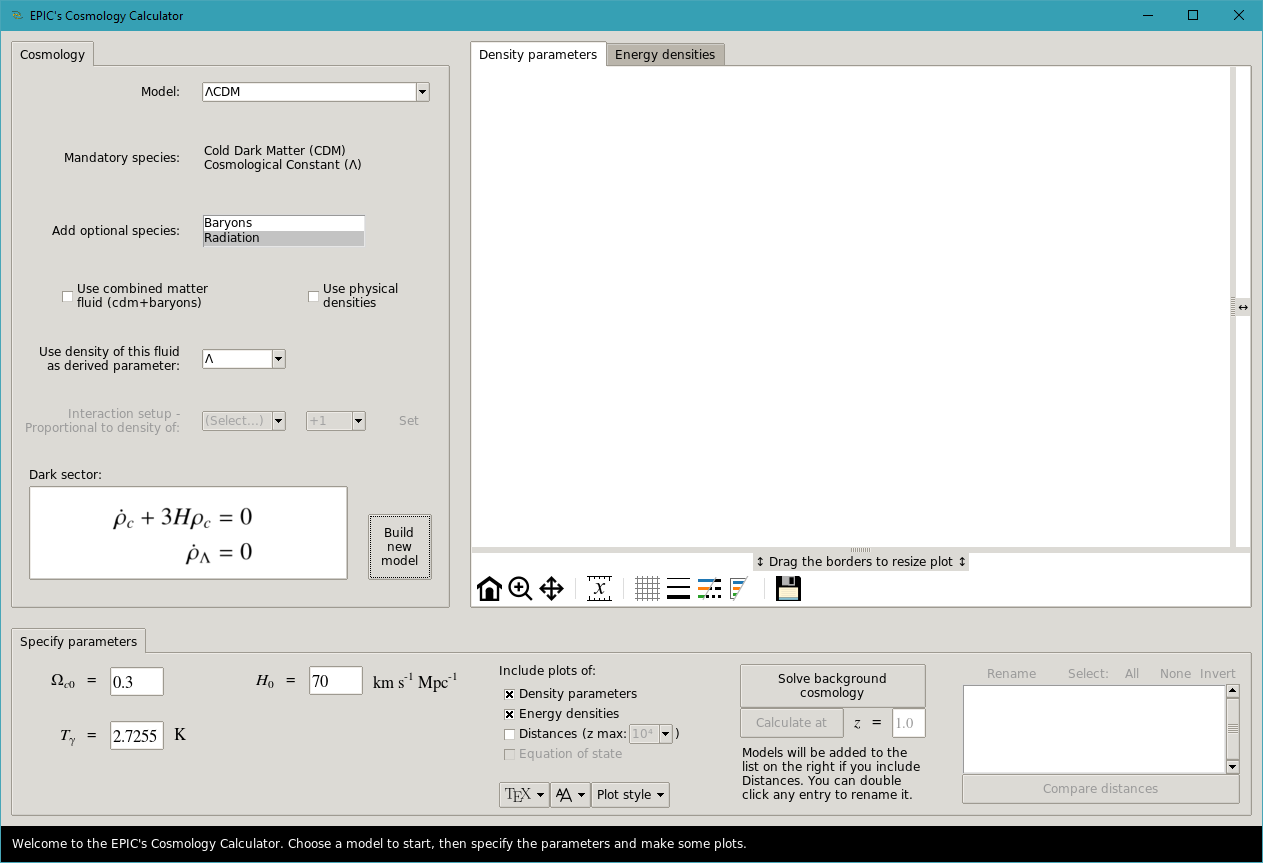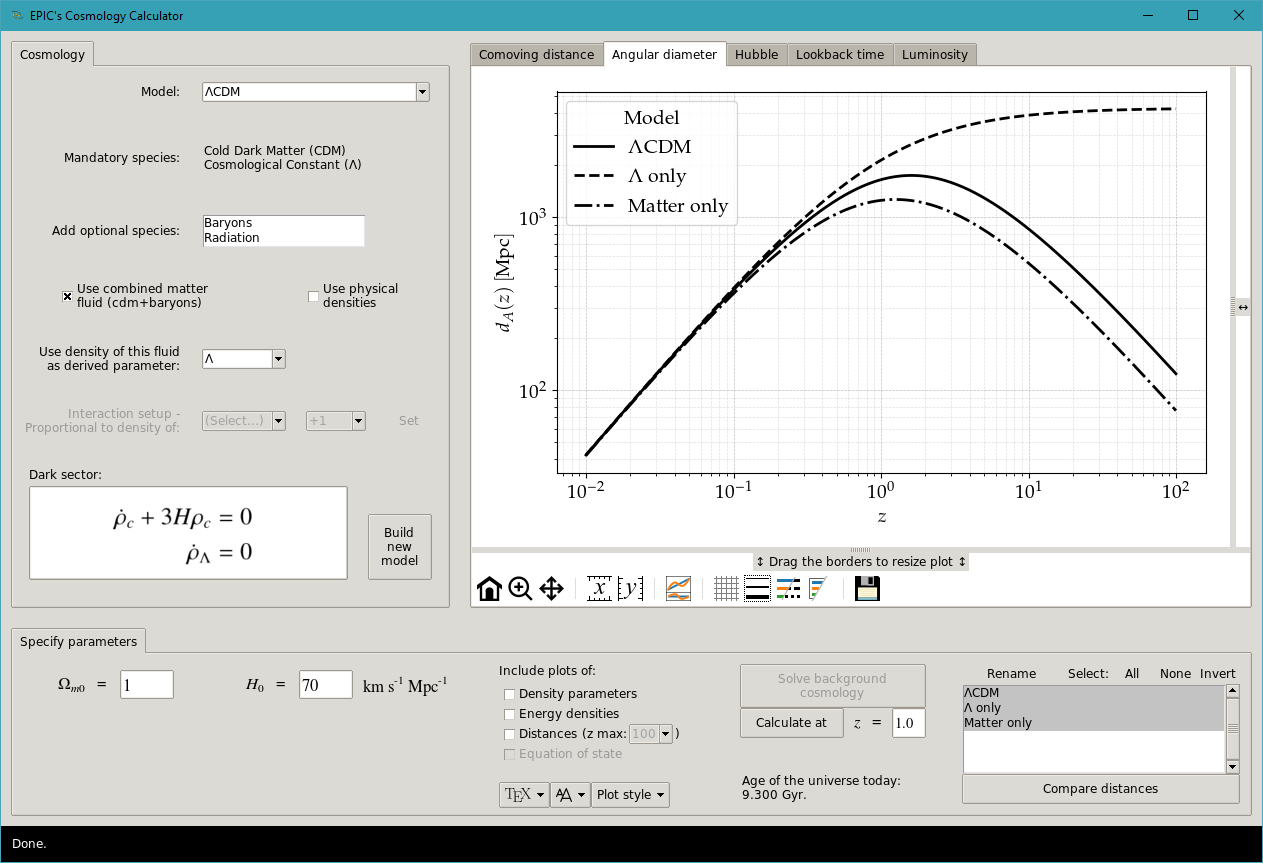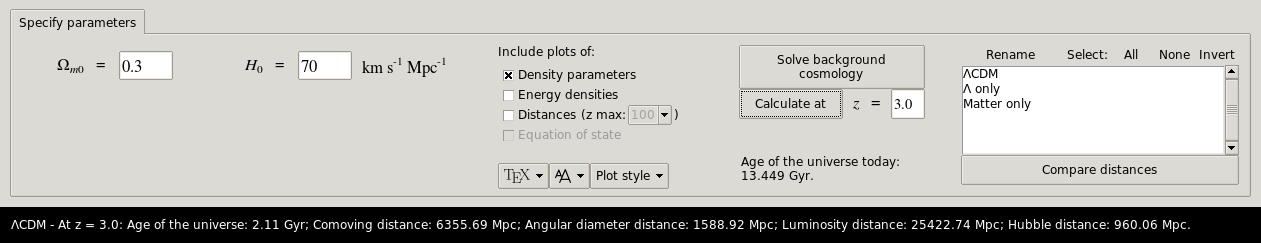# 3.4. The calculator GUI¶

Calculations like the ones presented in the previous section can also be executed from a Graphical User Interface (GUI). This makes it easy for the unexperienced user, although learning to use EPIC with the interactive Python interpreter is encouraged. The GUI can be launched with the command gui:

$python epic.py gui  Optionally, you can use $ python -W ignore epic.py gui to ignore some warnings that will be issued by matplotlib (this will actually omit all warnings). The following screen will appear:The tab in the left is where the cosmology is specified: you can choose one of the available models implemented. The required species will be listed. Below them, the optional fluids that the user can add just clicking them to select/deselect. You will always be able to choose whether or not to use physical densities. If the cold dark matter (CDM) component in the select model does not interact with dark energy, the option to combine CDM with baryons in a single matter fluid will be available, in which case the optional inclusion of baryons will be ignored. You can then choose which fluid’s density to express in terms of the others’, as a derived parameter, and set the interaction, if that is the case. The energy conservation equations for the dark sector in the given model configuration will be displayed in the box at the bottom of the frame. Click the “Build new model” button to proceed.In the “Specify parameters” section, the free parameters will be displayed together with entry fields where the use can change their values. Adjust them to your liking. The next section of controls allows you to choose which plots to display. EPIC will solve the cosmology and find the background energy densities and density parameters for all fluids. The calculation of distances over a wide range of redshifts is optional. With this option enabled, plots of the comoving distance $$\chi(z) = c \int_0^z \left[H(\tilde z)\right]^{-1} \mathrm{d}\tilde z$$, the angular diameter distance $$d_A$$, which is equal to $$a \chi$$ in the flat universe, the luminosity distance $$d_L = d_A / a^2$$, the Hubble distance $$d_H \equiv c/H(z)$$ and the lookback time $$t(z) = \int_0^z \left[\left(1+\tilde z\right)H(\tilde z) \right]^{-1} \mathrm{d}\tilde z$$ are generated. You can still customize the look of the plots with the menu buttons at the button. They let you choose between several styles from the matplotlib library, turn on the use of $$\LaTeX$$ for rendering text (which is slower), with a few typeface options and change the size of the text labels. These options can be changed at any moment – existing plots will be updated with these settings.

When you choose to include the calculation of distances, the model will be added to the list in the bottom right. With this, if you build other models you will be able to compare their distances between the different model configurations.

Note

If you want to add another instance of the same model (with different values of parameters) to see a comparison of distances, you need to click the “Build new model” button again, otherwise the previous model will be overwritten, since no new CosmologicalSetup object is created.

After the results for each model are presented, you can select the models that you wish to have their distances compared and click “Compare distances”.The comparison is shown for all the distances listed above. Besides the navigation controls, there are buttons in the toolbar below the plots that enable the inclusion or removal of a bottom frame showing the residual differences using the first model listed (i.e., amongst the selected ones) as the reference. In other cases, it will be possible to toggle the scale of the axes between logarithmic and linear. In all cases, you will be able to cycle through some options of grid, line widths, alternate between colored lines or different line styles, omit the legend’s title or the entire legend, and finally save the figure in pdf and png formats, together with the plotted data in txt files. The size of the plots can be adjusted using the handles prior to saving. Note that they affect only the plot currently shown. To resize all of them at the same time, try leaving them maximized with these controls and then resizing the app window.

## Calculating distances at a given redshift¶

After having computed the background solution for a given model, you can print the distances at any specified redshift using the button “Calculate at”. The results are printed in the status bar.